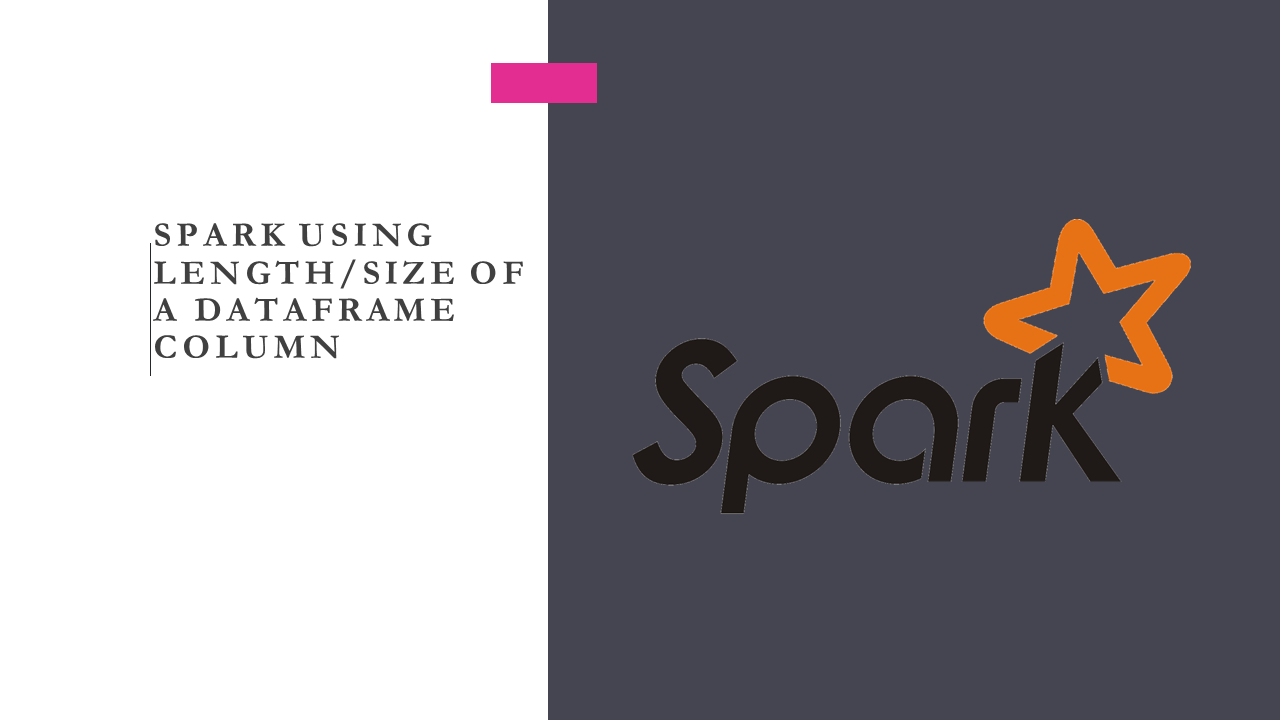# Spark Using Length/Size Of a DataFrame Column

Question: In Spark & PySpark is there a function to filter the DataFrame rows by length or size of a String Column (including trailing spaces) and also show how to create a DataFrame column with the length of another column.

## Solution: Filter DataFrame By Length of a Column

Spark SQL provides a` length()` function that takes the DataFrame column type as a parameter and returns the number of characters (including trailing spaces) in a string. This function can be used to filter() the DataFrame rows by the length of a column.

If the input column is Binary, it returns the number of bytes.

``````
val data = Seq(("James"),("Michael     "),("Robert  "))
import spark.sqlContext.implicits._
val df = data.toDF("name_col")
``````

## Spark Filter DataFrame by length Example

The below example filter/select the DataFrame rows that has character length greater then 5 on `name_col` column.

``````
//Filter DataFrame by checking the length of a column
import org.apache.spark.sql.functions.{col,length}
df.filter(length(col("name_col")) >5).show()

//+------------+
//|    name_col|
//+------------+
//|Michael     |
//|    Robert  |
//+------------+
``````

## Create a New Column with the length of a Another Column

Below example creates a new column ‘`len_col`‘ with the length of an existing column including trailing spaces, if you don’t want to include spaces, use trim() function to remove the spaces on the column before getting length().

``````
//Create new column with the length of existing string column
import org.apache.spark.sql.functions.{col,length,trim}
df.withColumn("len_col",length(col("name_col")))
.withColumn("trim_len_col",length(trim(col("name_col"))))
.show()

//+------------+-------+------------+
//|    name_col|len_col|trim_len_col|
//+------------+-------+------------+
//|       James|      5|           5|
//|Michael     |     12|           7|
//|    Robert  |      8|           6|
//+------------+-------+------------+
``````

Alternatively you can also write the same statement using select() transformation. This yields the same output as above.

``````
//Create new column with the length of existing string column
df.select(col("name_col"),
length(col("name_col")).alias("len_col"),
length(trim(col("name_col"))).alias("trim_len_col")
).show()
``````

## PySpark Example to Filter DataFrame by the length of a Column

In PySpark you can use the length() function by importing from `pyspark.sql.functions import length`.

``````
#Filter DataFrame by checking the length of a column
from pyspark.sql.functions import col,length,trim
df.filter(length(col("name_col")) >5).show()

#Create new column with the length of an existing string column
df.withColumn("len_col",length(col("name_col"))) \
.withColumn("trim_len_col",length(trim(col("name_col")))) \
.show()
``````

## Spark SQL Example Column Length

Similarly, you can also use the length() function on Spark SQL expression after creating temporary table from DataFrame.

In SQL, you can also use char_length() and character_length() functions to get the length of a string including trailing spaces.

``````
//Using length() function on SQL
df.createOrReplaceTempView("TAB")
spark.sql("select name_col,length(name_col) as len_col " +
"from TAB where length(name_col) > 5").show()

//+------------+-------+
//|    name_col|len_col|
//+------------+-------+
//|Michael     |     12|
//|    Robert  |      8|
//+------------+-------+
``````

## Conclusion

How to Filter the DataFrame rows by using length/size of the column is frequently asked question in Spark & PySpark, you can do this by using the length() SQL function, this function considers trailing spaces into the size, if you wanted to remove spaces use trim() function with length().

Happy Learning !!

### Naveen (NNK)

Naveen (NNK) is a Data Engineer with 20+ years of experience in transforming data into actionable insights. Over the years, He has honed his expertise in designing, implementing, and maintaining data pipelines with frameworks like Apache Spark, PySpark, Pandas, R, Hive and Machine Learning. Naveen journey in the field of data engineering has been a continuous learning, innovation, and a strong commitment to data integrity. In this blog, he shares his experiences with the data as he come across. Follow Naveen @ @ LinkedIn